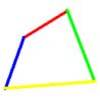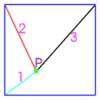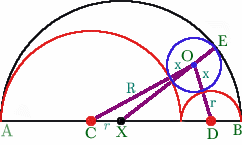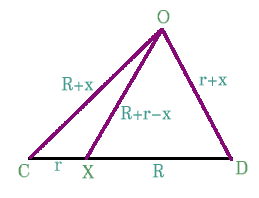#### You may also like### Biggest Bendy

Four rods are hinged at their ends to form a quadrilateral. How can you maximise its area?### Square World

P is a point inside a square ABCD such that PA= 1, PB = 2 and PC = 3. How big is angle APB ?### 30-60-90 Polypuzzle

Re-arrange the pieces of the puzzle to form a rectangle and then to form an equilateral triangle. Calculate the angles and lengths.

# Just Touching

##### Age 16 to 18Challenge Level

This solution comes from Sue Liu of Madras CollegeLet the large semi-cirle have diameter $AB$ and centre $X$. Let the two smaller semi-circles have centres $C$ and $D$ and radii $R$ and $r$. Thus $AC = R$, $BD = r$ so that $AX = (2R + 2r)/2 = (R + r)$ and $CX = r$ from which it follows that $XD = R$.

Let the small circle have have centre $O$ and radius $x$. Then $CO = R + x$ and $DO = r + x$. The line $XO$, joining the centre of the large semicircle to the centre of the small circle, cuts the circumference of the large semicircle at $E$ where $XE = XB = R + r$, $OE = x$ and $OX = R + r - x$.

If we now consider the triangle $OCD$ we have$$\angle OXC + \angle OXD = 180^o$$
So
\begin{eqnarray} \\ \cos \angle OXC + \cos \angle OXD &=& 0 \\ \frac{r^2 + (R + r - x)^2 - (R + x)^2}{2r(R + r - x)} + \frac{R^2 + (R + r - x)^2 - (r + x)^2}{2R(R + r - x)} &=& 0 \\ r^2R + R(R + r - x)^2 - R(R + x)^2 + rR^2 + r(R + r - x)^2 - r(r + x)^2 &=& 0 \\ 4R^2r +4Rr^2 &=& 4xR^2 + 4xr^2 + 4Rrx \\ R^2r + Rr^2 &=& x(R^2 + rR + r^2) \\ x &=& \frac{Rr(R + r)}{R^2 + Rr + r^2} \end{eqnarray}

There is a pleasing symmetry about this formula which gives the radius of the small circle in terms of the radii of the two smaller semicircles. An excellent solution, well done Sue!